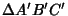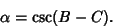## Kiepert's Parabola

Let three similar Isosceles Triangles,, andbe constructed on the sides of a Triangle. Then the Envelope of the axis of the Trianglesandis Kiepert's parabola, given by(1)(2)

whereare the Trilinear Coordinates for a line tangent to the parabola. It is tangent to the sides of the Triangle, the line at infinity, and the Lemoine Line. The Focus has Triangle Center Function(3)

The Euler Line of a triangle is the Directrix of Kiepert's parabola. In fact, the Directrices of all parabolas inscribed in a Triangle pass through the Orthocenter. The Brianchon Point for Kiepert's parabola is the Steiner Point.

See also Brianchon Point, Envelope, Euler Line, Isosceles Triangle, Lemoine Line, Steiner Points